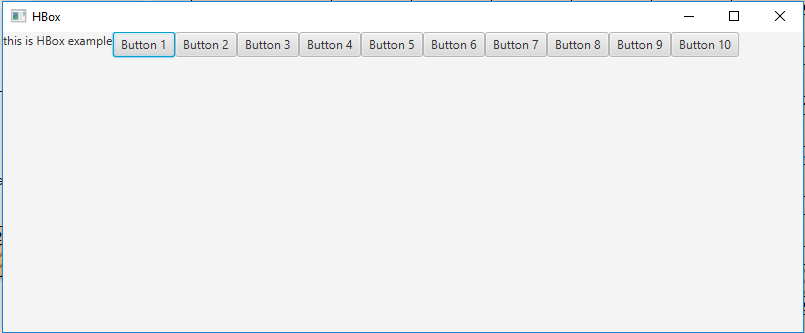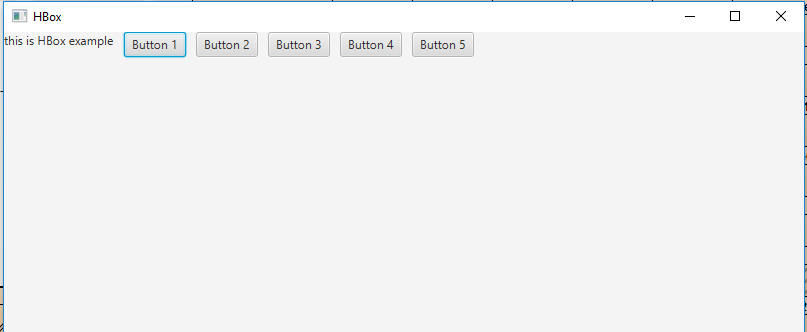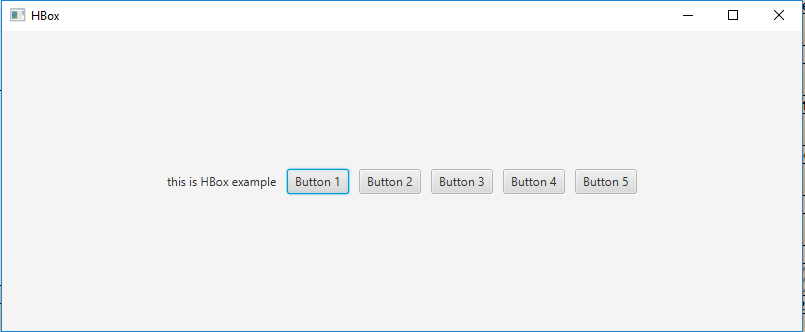# JavaFX | HBox Class

HBox is a part of JavaFX. HBox lays out its children in form of horizontal columns. If the HBox has a border and/or padding set, then the contents will be layed out within those insets. HBox class extends Pane class.

Constructors of the class:

• HBox(): Creates an HBox object with no nodes.
• HBox(double s): Creates an HBox with spacing in between nodes.

Commonly Used Methods:

Method Explanation
getAlignment() Returns the value of property alignment.
getSpacing() Returns the spacing between its children.
setAlignment(Pos value) Sets the Alignment of the HBox.
getChildren() Returns the nodes in HBox.

Below programs illustrate the use of HBox class:

1. Java Program to create a HBox and add it to the stage: In this program we will create a HBox named hbox. Now create a label and add it to the hbox. We will also create some buttons and add them to the HBox using the getChildren().add() function. Now create a scene and add the hbox to the scene and add the scene to the stage and call show() function to display the final results.

 `// Java Program to create a HBox ` `// and add it to the stage ` `import` `javafx.application.Application; ` `import` `javafx.scene.Scene; ` `import` `javafx.scene.control.*; ` `import` `javafx.scene.layout.*; ` `import` `javafx.stage.Stage; ` `import` `javafx.event.ActionEvent; ` `import` `javafx.event.EventHandler; ` `import` `javafx.scene.canvas.*; ` `import` `javafx.scene.web.*; ` `import` `javafx.scene.Group; ` ` `  `public` `class` `HBOX_1 ``extends` `Application { ` ` `  `    ``// launch the application ` `    ``public` `void` `start(Stage stage) ` `    ``{ ` ` `  `        ``try` `{ ` ` `  `            ``// set title for the stage ` `            ``stage.setTitle(``"HBox"``); ` ` `  `            ``// create a HBox ` `            ``HBox hbox = ``new` `HBox(); ` ` `  `            ``// create a label ` `            ``Label label = ``new` `Label(``"this is HBox example"``); ` ` `  `            ``// add label to hbox ` `            ``hbox.getChildren().add(label); ` ` `  `            ``// add buttons to HBox ` `            ``for` `(``int` `i = ``0``; i < ``10``; i++)  ` `            ``{ ` `                ``hbox.getChildren().add(``new` `Button(``"Button "`  `                                           ``+ (``int``)(i + ``1``))); ` `            ``} ` ` `  `            ``// create a scene ` `            ``Scene scene = ``new` `Scene(hbox, ``800``, ``300``); ` ` `  `            ``// set the scene ` `            ``stage.setScene(scene); ` ` `  `            ``stage.show(); ` `        ``} ` ` `  `        ``catch` `(Exception e) { ` ` `  `            ``System.out.println(e.getMessage()); ` `        ``} ` `    ``} ` ` `  `    ``// Main Method ` `    ``public` `static` `void` `main(String args[]) ` `    ``{ ` ` `  `        ``// launch the application ` `        ``launch(args); ` `    ``} ` `} `

Output:2. Java Program to create a HBox, add spaces between its elements and add it to the stage: In this program we will create a HBox named hbox. Set the spacing by passing a double value of space as an argument to the constructor. Now create a label and add it to the hbox. To add some buttons to the HBox use the getChildren().add() function. Finally, create a scene and add the hbox to the scene and add the scene to the stage and call show() function to display the final results.

 `// Java Program to create a HBox, add ` `// spaces between its elements and add ` `// it to the stage ` `import` `javafx.application.Application; ` `import` `javafx.scene.Scene; ` `import` `javafx.scene.control.*; ` `import` `javafx.scene.layout.*; ` `import` `javafx.stage.Stage; ` `import` `javafx.event.ActionEvent; ` `import` `javafx.event.EventHandler; ` `import` `javafx.scene.canvas.*; ` `import` `javafx.scene.web.*; ` `import` `javafx.scene.Group; ` ` `  `public` `class` `HBOX_2 ``extends` `Application { ` ` `  `    ``// launch the application ` `    ``public` `void` `start(Stage stage) ` `    ``{ ` ` `  `        ``try` `{ ` ` `  `            ``// set title for the stage ` `            ``stage.setTitle(``"HBox"``); ` ` `  `            ``// create a HBox ` `            ``HBox hbox = ``new` `HBox(``10``); ` ` `  `            ``// create a label ` `            ``Label label = ``new` `Label(``"this is HBox example"``); ` ` `  `            ``// add label to hbox ` `            ``hbox.getChildren().add(label); ` ` `  `            ``// add buttons to HBox ` `            ``for` `(``int` `i = ``0``; i < ``5``; i++)  ` `            ``{ ` `                ``hbox.getChildren().add(``new` `Button(``"Button "`  `                                          ``+ (``int``)(i + ``1``))); ` `            ``} ` ` `  `            ``// create a scene ` `            ``Scene scene = ``new` `Scene(hbox, ``800``, ``300``); ` ` `  `            ``// set the scene ` `            ``stage.setScene(scene); ` ` `  `            ``stage.show(); ` `        ``} ` ` `  `        ``catch` `(Exception e) { ` ` `  `            ``System.out.println(e.getMessage()); ` `        ``} ` `    ``} ` ` `  `    ``// Main Method ` `    ``public` `static` `void` `main(String args[]) ` `    ``{ ` ` `  `        ``// launch the application ` `        ``launch(args); ` `    ``} ` `} `

Output:3. Java Program to create a HBox, add spaces between its elements, set an alignment and add it to the stage: In this program we will create a HBox named hbox. Set the spacing by passing a double value of space as an argument to the constructor. Set the alignment of the HBox using the setAlignment() function. Then create a label and add it to the hbox. Add some buttons to the HBox using the getChildren().add() function. Finally, create a scene and add the hbox to the scene and add the scene to the stage and call show() function to display the final results.

 `// Java Program to create a HBox, add spaces ` `// between its elements, set an alignment ` `// and add it to the stage ` `import` `javafx.application.Application; ` `import` `javafx.scene.Scene; ` `import` `javafx.scene.control.*; ` `import` `javafx.scene.layout.*; ` `import` `javafx.stage.Stage; ` `import` `javafx.event.ActionEvent; ` `import` `javafx.event.EventHandler; ` `import` `javafx.scene.canvas.*; ` `import` `javafx.scene.web.*; ` `import` `javafx.scene.Group; ` `import` `javafx.geometry.*; ` ` `  `public` `class` `HBOX_3 ``extends` `Application { ` ` `  `    ``// launch the application ` `    ``public` `void` `start(Stage stage) ` `    ``{ ` ` `  `        ``try` `{ ` ` `  `            ``// set title for the stage ` `            ``stage.setTitle(``"HBox"``); ` ` `  `            ``// create a HBox ` `            ``HBox hbox = ``new` `HBox(``10``); ` ` `  `            ``// setAlignment ` `            ``hbox.setAlignment(Pos.CENTER); ` ` `  `            ``// create a label ` `            ``Label label = ``new` `Label(``"this is HBox example"``); ` ` `  `            ``// add label to hbox ` `            ``hbox.getChildren().add(label); ` ` `  `            ``// add buttons to HBox ` `            ``for` `(``int` `i = ``0``; i < ``5``; i++)  ` `            ``{ ` `                ``hbox.getChildren().add(``new` `Button(``"Button "`  `                                          ``+ (``int``)(i + ``1``))); ` `            ``} ` ` `  `            ``// create a scene ` `            ``Scene scene = ``new` `Scene(hbox, ``800``, ``300``); ` ` `  `            ``// set the scene ` `            ``stage.setScene(scene); ` ` `  `            ``stage.show(); ` `        ``} ` ` `  `        ``catch` `(Exception e) { ` ` `  `            ``System.out.println(e.getMessage()); ` `        ``} ` `    ``} ` ` `  `    ``// Main Method ` `    ``public` `static` `void` `main(String args[]) ` `    ``{ ` ` `  `        ``// launch the application ` `        ``launch(args); ` `    ``} ` `} `

Output:Note: The above programs might not run in an online IDE please use an offline compiler.

My Personal Notes arrow_drop_upSecond year Department of Information Technology Jadavpur University

If you like GeeksforGeeks and would like to contribute, you can also write an article using contribute.geeksforgeeks.org or mail your article to contribute@geeksforgeeks.org. See your article appearing on the GeeksforGeeks main page and help other Geeks.

Please Improve this article if you find anything incorrect by clicking on the "Improve Article" button below.

Article Tags :
Practice Tags :

Be the First to upvote.

Please write to us at contribute@geeksforgeeks.org to report any issue with the above content.